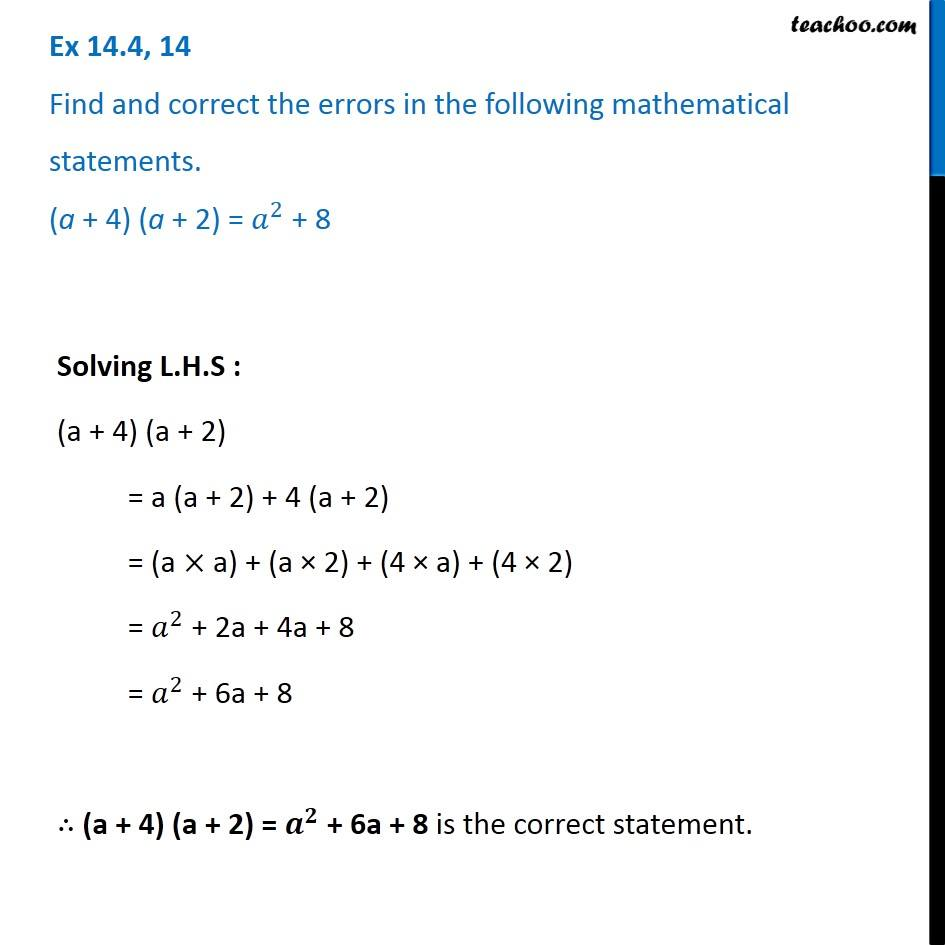Identify and correct errors in the sentences

Chapter 12 Class 8 Factorisation
Serial order wiseLearn in your speed, with individual attention - Teachoo Maths 1-on-1 Class

### Transcript

Question 14 Find and correct the errors in the following mathematical statements. (a + 4) (a + 2) = 𝑎^2 + 8 Solving L.H.S : (a + 4) (a + 2) = a (a + 2) + 4 (a + 2) = (a × a) + (a × 2) + (4 × a) + (4 × 2) = 𝑎^2 + 2a + 4a + 8 = 𝑎^2 + 6a + 8 ∴ (a + 4) (a + 2) = 𝒂^𝟐 + 6a + 8 is the correct statement.